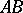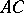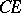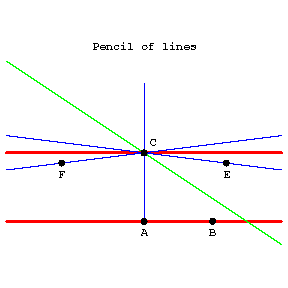# Parallel straight lines

(diff) ← Older revision | Latest revision (diff) | Newer revision → (diff)

in Euclidean geometry

Lines that lie in one plane and that do not intersect. In absolute geometry there passes through a point not lying on a given line at least one line that does not intersect the given one. In Euclidean geometry there is only one such line. This fact is equivalent to Euclid's fifth postulate (on parallels). In Lobachevskii geometry in the plane there pass through a point(see Fig.) outside a given lineinfinitely-many lines that do not intersect. Of these only two are called parallel to. A lineis said to be parallel toin the direction fromtoif: 1)andlie on the same side of; 2)does not intersect; and 3) every ray within the angleintersects the line. The lineparallel toin the direction fromtois defined similarly.Figure: p071380a